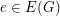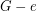# 3-Edge-Coloring Conjecture

 Importance: High ✭✭✭
 Author(s): Arthur Hoffmann-Ostenhof
 Subject: Graph Theory
 Keywords: 3-edge coloring 4-flow removable edge
Conjecture   Supposewithis a connected cubic graph admitting a-edge coloring. Then there is an edgesuch that the cubic graph homeomorphic tohas a-edge coloring.
Conjecture   Supposeis a cubic graph with a nowhere-zero-flow, then there is an edgesuch thathas a nowhere-zero-flow.